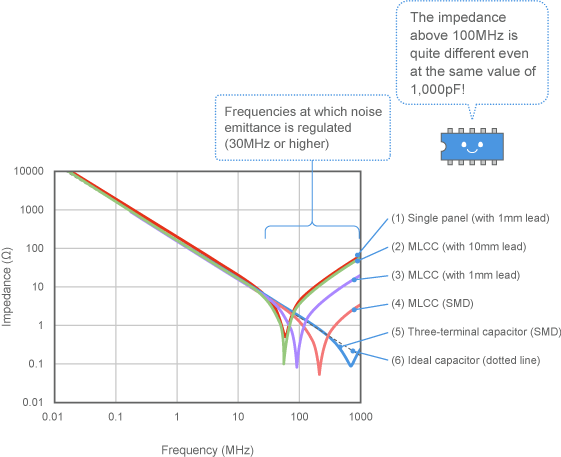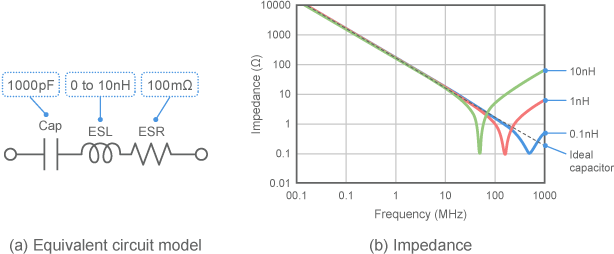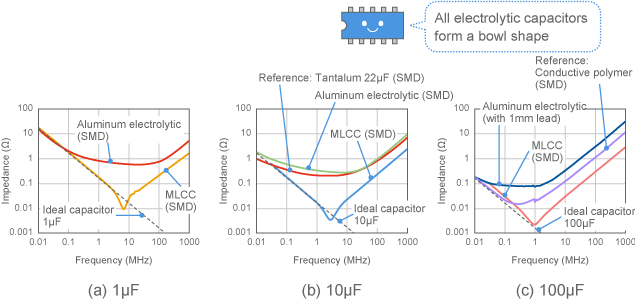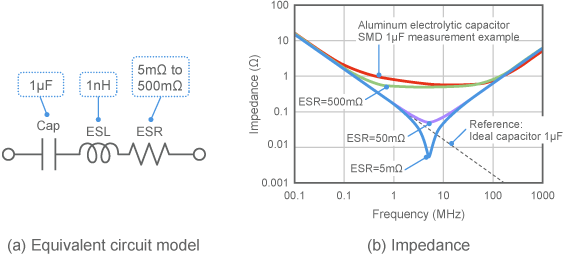# Noise Suppression Products/EMI Suppression Filters

Noise Suppression Basic Course Section 1
Chapter 1
Chapter 2
Chapter 3
Chapter 4
Chapter 5
Chapter 6
Chapter 6

## EMI suppression filters

#### 6-5-3. Effect of capacitor parasitic elements

##### (1) How does impedance change?

The previous section introduced the fact that a capacitor's impedance frequency characteristics form a V-shape, and that low frequencies (left side) and high frequencies (right side) correspond to electrostatic capacitance and ESL, respectively. You can easily control the electrostatic capacitance of a capacitor by specifying a part number. How much of an effect does ESL have?
Fig. 12 shows an example of the impedance measured from several types of ceramic capacitors with a nominal electrostatic capacitance of 1,000pF. The figure shows that...

1. (a) MLCC (laminate structure)  (rather than a single board)
2. (b) Capacitors with shorter leads
3. (c) SMD capacitors (rather than capacitors with leads)

are all closer to the ideal capacitor and have less impedance up until the higher frequencies. This also demonstrates that ESL decreases in this order. This tendency is generally seen in all capacitors—not only in ceramic capacitors. This is because the main factors behind ESL are the internal electrode and lead shapes.
When using a capacitor to eliminate emitted noise, it will be used at a frequency of 30MHz or higher. As shown in the figure, there can be a tenfold difference or more at this frequency due to differences in ESL, even if the same 1,000pF capacitor is used.Fig. 12 Example of changes in impedance when installation is modified (1,000pF)

##### (2) What is the value of ESL?

So what will be the value of ESL now?
Figure 13 shows the results of calculating the impedance after changing the ESL, on a 1,000pF capacitor using an equivalent circuit model. Comparing Fig. 12 and Fig. 13, we can estimate that ESL will be

• Around 10nH for an MLCC with a 10mm lead ( (2) in Fig. 12)
• 1nH or less for an SMD MLCC with no lead ( (4) in Fig. 12)
• 0.1nH or less for a three-terminal capacitor ( (5) in Fig. 12)

The nH values mentioned here are extremely small values that occur even on a lead that is only several mm long. Looking at the frequencies above 100MHz on the graph shows that even this small amount of inductance has a significant effect.
Note that the three-terminal capacitor shown in (5) in Fig. 12 is a high performance capacitor with a special structure meant to reduce ESL. Three-terminal capacitors will be further described in Chapter 8.Fig. 13 Results of calculating impedance when ESL is changed

##### (3) Use with as short a capacitor lead as possible

It is better to use a capacitor with little ESL to suppress noise. As shown in (2) , (3) , and (4) in Fig. 12, the lead should be as short as possible when using a capacitor (and should be SMD if possible) .
Actually, during the experiment shown in Fig. 2 in Section 6.4, the noise reduction effect was changed using the differences in the ESL of the capacitors themselves, as well as the ESL differences caused by whether or not a lead was present. If a capacitor is installed over an approximately 10mm long lead (Section 6.4, Fig. 2 (d) ) , the noise reduction effect will be at least 10dB less compared to a scenario with no lead (Section 6.4, Fig. 2 (c) ) .

##### (4) Impedance characteristics of electrolytic capacitors

The explanation of capacitor characteristics up until now has mostly used MLCCs as an example. For applications requiring a large electrostatic capacitance (such as power leveling), electrolytic capacitors with a large electrostatic capacitance per volume are used. The impedance characteristics of electrolytic capacitors differ slightly from those of MLCCs. Fig. 14 shows some comparison examples.
Aluminum electrolytic capacitors are sometimes used for power leveling. Fig. 14 shows that the impedance curve of an aluminum electrolytic capacitor forms a bowl shape (or U shape) . It also shows that self-resonance cannot be clearly seen. This means that the capacitor loss is comparatively large; there will be a significant ESR in the equivalent circuit in Fig. 7.Fig. 14 Examples comparing electrolytic capacitor and MLCC impedance

##### (5) What effect does ESR have?

Fig. 15 shows the results of calculating the impedance when ESR is changed, with a 1μF capacitor as an example. With ESR at 500 megaohms, it is possible to obtain characteristics similar to the results of measuring the aluminum electrolytic capacitor in Fig. 14 (a) . The impedance characteristics of an electrolytic capacitor can thus be reproduced by increasing ESR. The impedance corresponding to the bottom of the bowl-shaped characteristic curve represents the ESR value.
The ESR of an aluminum electrolytic capacitor can reach up to 1 ohm or more. The impedance of the capacitor will never be less than that the ESR; this means that a capacitor with a large ESR is unsuitable for use in noise suppression.
On the other hand, there are cases where a capacitor used to suppress noise will create resonance with the surrounding circuit, causing a malfunction. In this case, ESR can function as a resonance damping resistor to prevent this malfunction from happening. In this case, then, a capacitor with a somewhat large ESR would be of benefit.Fig. 15 Results of calculating changes in impedance when ESR is changed

##### (6) Electrolytic capacitors with low ESR

Some electrolytic capacitors are designed so that ESR is minimized. Examples include tantalum capacitors and conductive polymer capacitors. The measurement results in Fig. 14 also include examples using these capacitors. The figure shows that the impedance around the resonant frequency is smaller than that of an aluminum electrolytic capacitor.
However, this does not extend to MLCCs, even with these capacitors. A large capacity MLCC should be selected, or an MLCC should be added in parallel with an electrolytic capacitor and used, for cases where noise reduction is important even in applications that require a large electrostatic capacitance (such as power leveling) .

Top of page